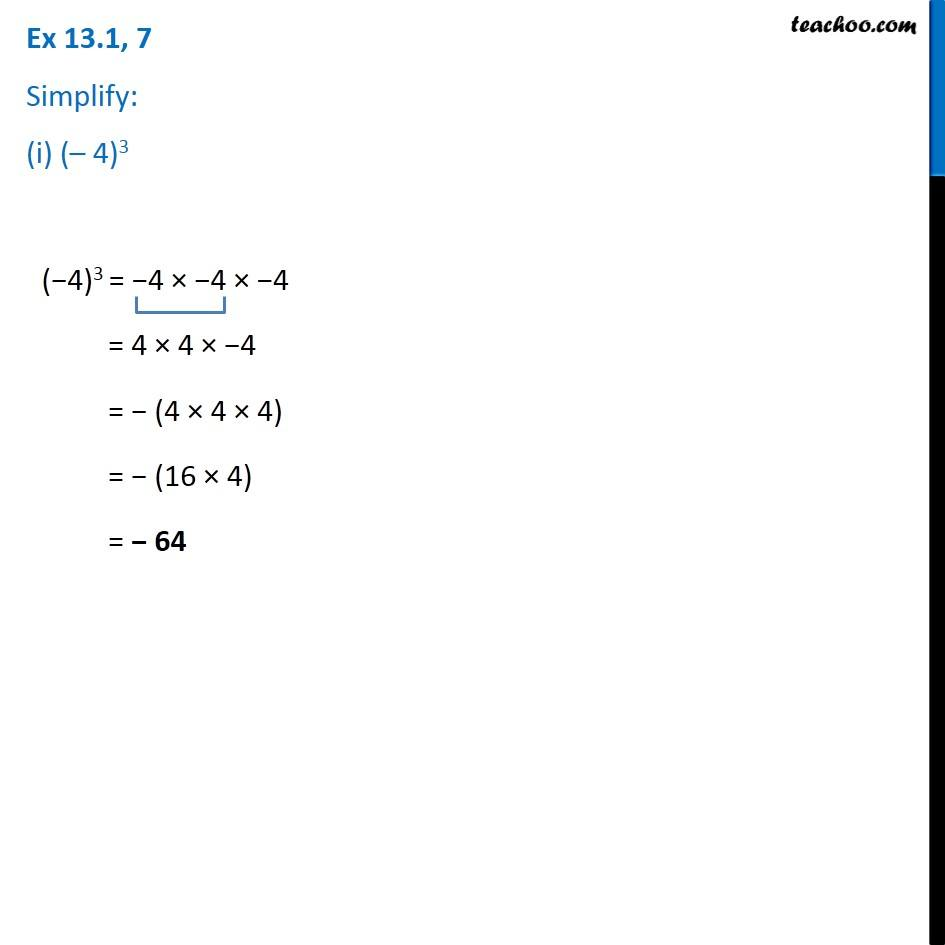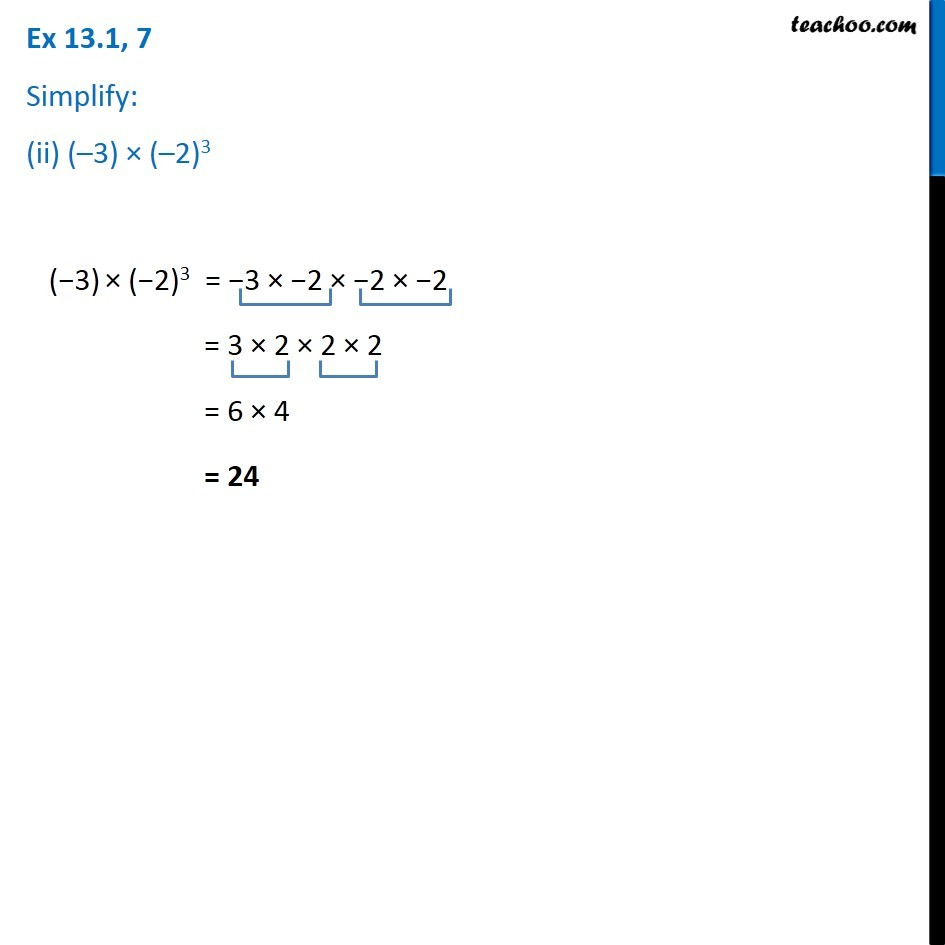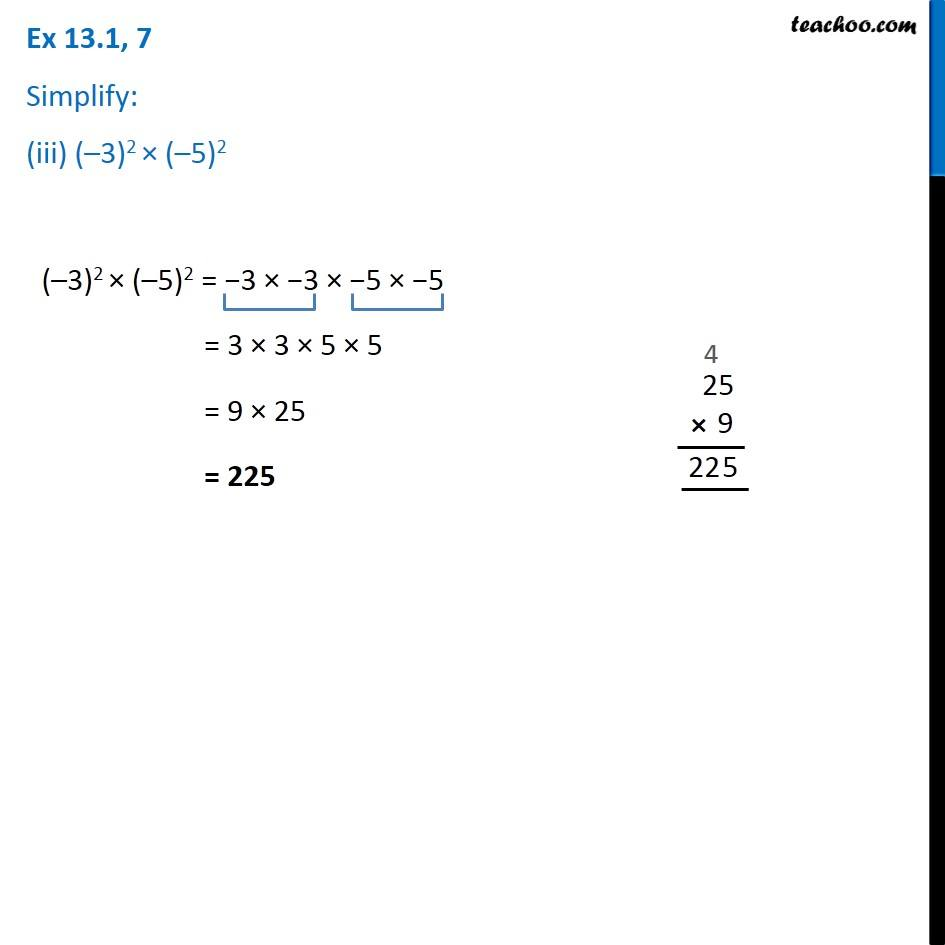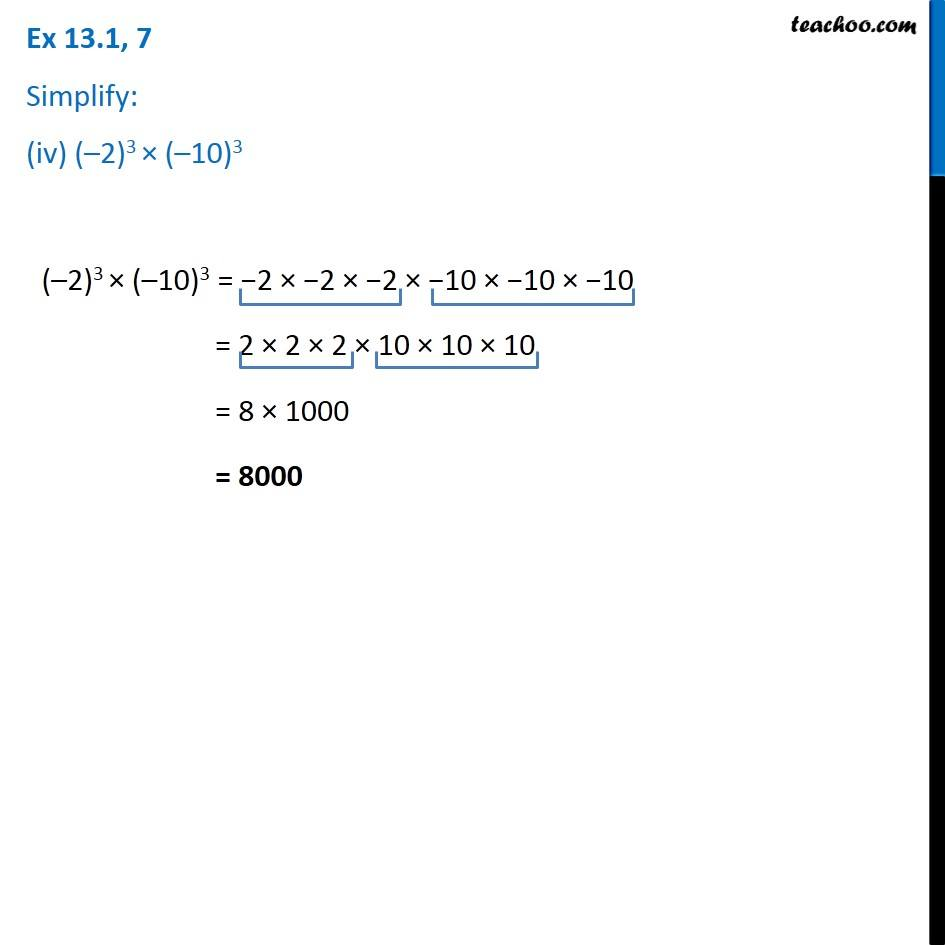1. Chapter 13 Class 7 Exponents and Powers
2. Serial order wise
3. Ex 13.1

Transcript

Ex 13.1, 7 Simplify: (i) (– 4)3 (−4)3 = −4 × −4 × −4 = 4 × 4 × −4 = − (4 × 4 × 4) = − (16 × 4) = − 64 Ex 13.1, 7 Simplify: (ii) (–3) × (–2)3 (−3) × (−2)3 = −3 × −2 × −2 × −2 = 3 × 2 × 2 × 2 = 6 × 4 = 24 Ex 13.1, 7 Simplify: (iii) (–3)2 × (–5)2 (–3)2 × (–5)2 = −3 × −3 × −5 × −5 = 3 × 3 × 5 × 5 = 9 × 25 = 225 Ex 13.1, 7 Simplify: (iv) (–2)3 × (–10)3 (–2)3 × (–10)3 = −2 × −2 × −2 × −10 × −10 × −10 = 2 × 2 × 2 × 10 × 10 × 10 = 8 × 1000 = 8000